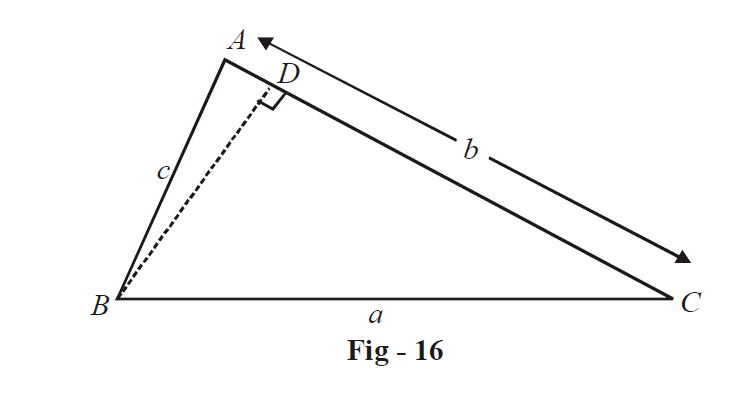# Cosine Rule

Go back to  'Trigonometry'

### Property - 2: Cosine rule

We use standard rotation. In a  $$\Delta ABC$$ ,

\begin{align}& {{a}^{2}}={{b}^{2}}+{{c}^{2}}-2bc\ \cos A \\ & {{b}^{2}}={{c}^{2}}+{{a}^{2}}-2ac\ \cos B \\ & {{c}^{2}}={{a}^{2}}+{{b}^{2}}-2ab\ \cos C \end{align}

The justification is trivial. We consider the first part.\begin{align}&{\text{We have,}} \\ & {a^2} = B{D^2} + C{D^2} \\ \;\;\;\; &\quad= \;{\left( {c\sin A} \right)^2} + {\left( {AC - AD} \right)^2} \\ \;\;\;\; &\quad= {\left( {c\sin A} \right)^2} + {\left( {b - c\cos A} \right)^2} \\ \;\;\;\; &\quad= {b^2} + {c^2} - 2bc\cos A \\ \end{align}

Observe that these relations will hold even when the triangle is obtuse. If the triangle is right angled, say at A, the cosine rule reduces to the Pythagoras theorem $${a^2} = {b^2} + {c^2}$$